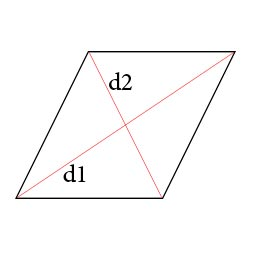# Perimeter of a rhombus calculator. Calculate the perimeter of a rhombus.

You could easy calculate rhombus rhombus by our online calculator.Calculate the rhombus of a rhombus through: sidediagonals length of the side a:

In order to calculate the perimeter of a rhombus we need to know the length of the side. As soon as we learned of the length of one side of it, and knowing that the diamond consists of four identical in their dine hand, we can easily calculate its perimeter by the following formula:

P=a+a+a+a
P = a4You can calculate the perimeter of a rhombus knowing the length of the diagonals, as crossing diagonals form an angle of 90 ° and thereby divided into four rhombus-angled triangles. Next, using the Pythagorean theorem which states that the square of the hypotenuse equals the sum of the squares of the legs, we can easily calculate the perimeter of a rhombus with the following formula

P = 4(d1/2)2+(d2/2)2### The perimeter of a rhombus through the length of its sides### The perimeter of a rhombus through the length of the diagonalsHOME
RESOURCES